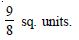# NCERT Solutions for Class 12 Mathemetics Chapter 8 - Applications of the Integrals

##### Question 1:

Find the area of the region bounded by the curve y2 = x and the lines x = 1, x = 4 and the x-axis.

y2 = x is right-handed parabola.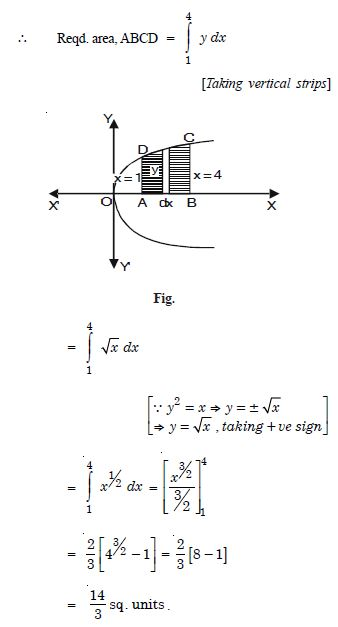##### Question 2:

Find the area of the region bounded by y2 = 9x, x = 2, x = 4 and the x-axis in the first quadrant.

The given parabola is y2 = 9x ..(1)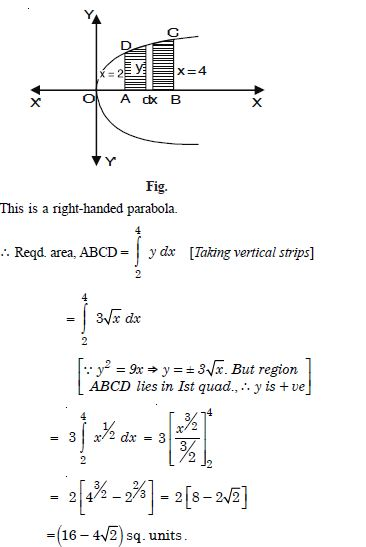##### Question 3:

Find the area of the region bounded by x2 = 4y, y = 2, y = 4 and the y-axis in the first quadrant.

x2 = 4y dx is an upward parabola.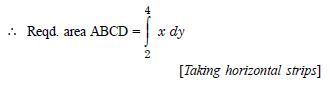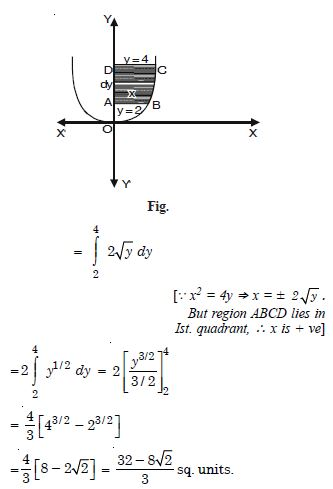##### Question 4: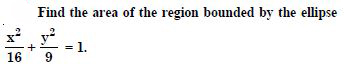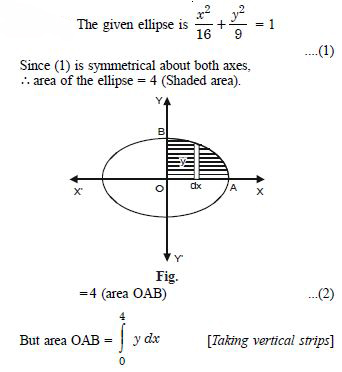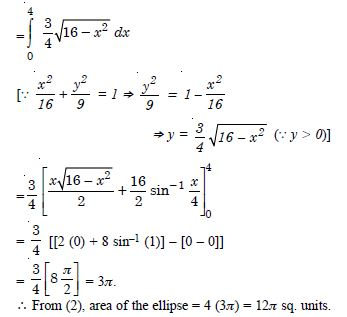##### Question 5: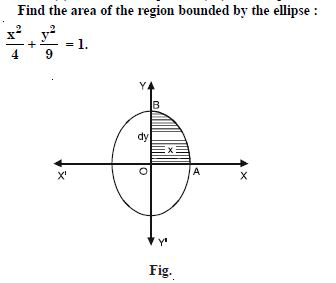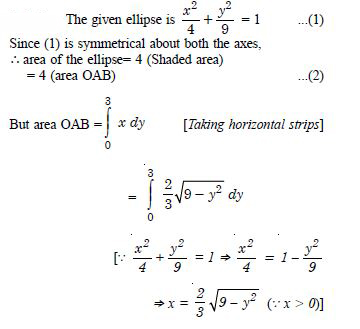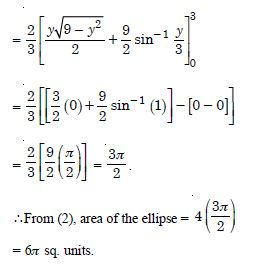##### Question 6: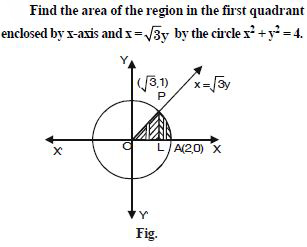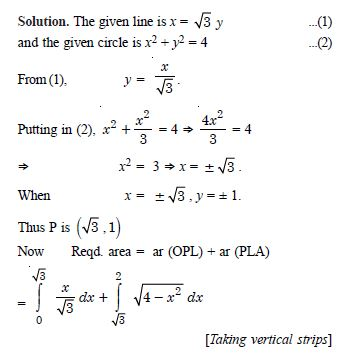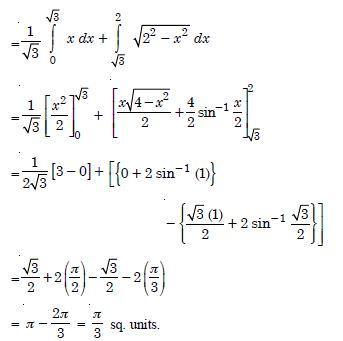##### Question 7: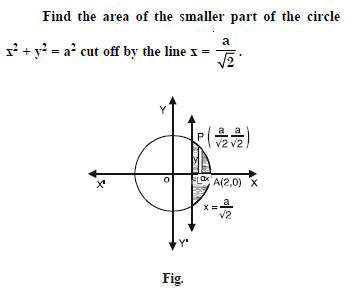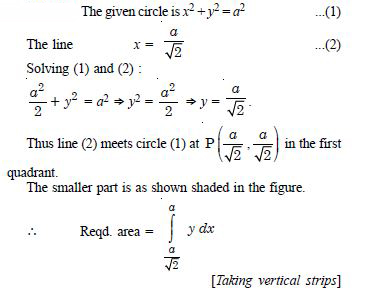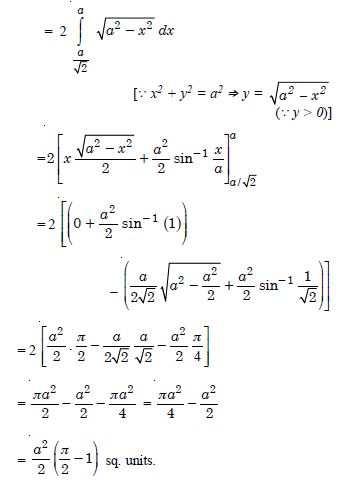##### Question 8:

The area between x = y2 and x = 4 is divided into two equal parts by the line x = a, find the value of a.

We have : x = y2 ....(1)
and x = 4 ...(2)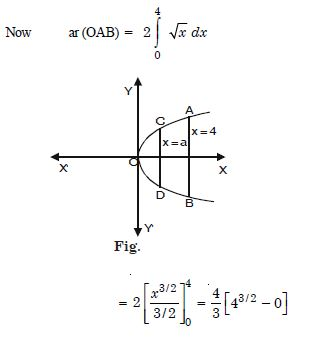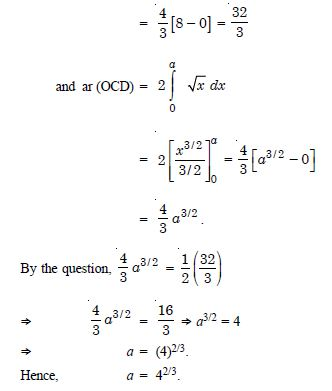##### Question 9:

Find the area of the region bounded by the parabola y = x2 and y = | x |.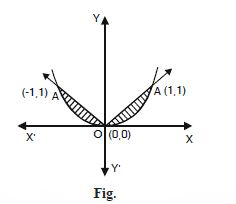The given parabola is x2 = y ...(1)
This is an upward parabola with vertex (0, 0).
y = | x | represents the st. lines :
y = x and y = –x ...(2)
y = x meets (1) at O (0, 0) and A (1, 1)
y = – x meets (1) at O (0, 0) and A¢ (– 1, 1).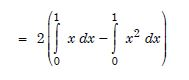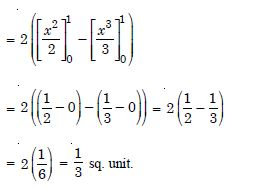##### Question 10:

Find the area bounded by the curve x2 = 4y and the line x = 4y – 2.

The given curve is x2 = 4y ...(1),
which is an upward parabola with vertex (0, 0).
The given line is x = 4y – 2 ...(2)
Solving (1) and (2) :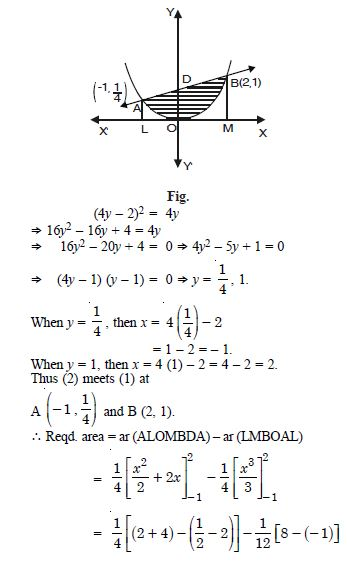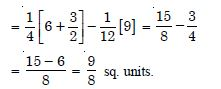##### Question 11:

Find the area of the region bounded by the curve y2 = 4x and the line x = 3.

The given curve is y2 = 4x ...(1)
and the given line is x = 3 ...(2)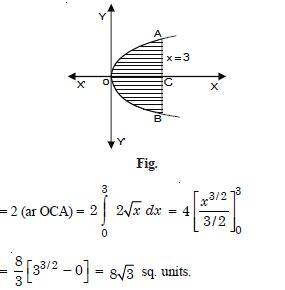##### Question 12:

Area lying in the first quadrant and bounded by the circle x2 + y2 = 4 and the lines x = 0 and x = 2 is :

1. $\mathrm{\pi }$
2.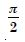3.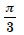4.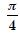$\mathrm{\pi }$

##### Question 13:

Area of the region bounded by the curve y2 = 4x, y-axis and the line y = 3 is :

1. 2
2.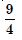3.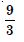4.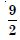##### Question 14:

Find the area of the circle 4x2 + 4y2 = 9, which is interior to the parabola x2 = 4y.

The given circle is 4x2 + 4y2 = 9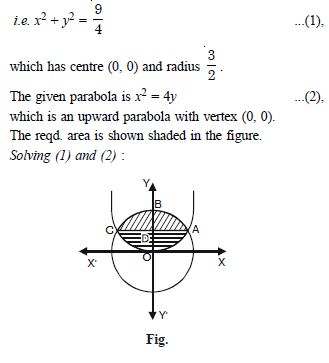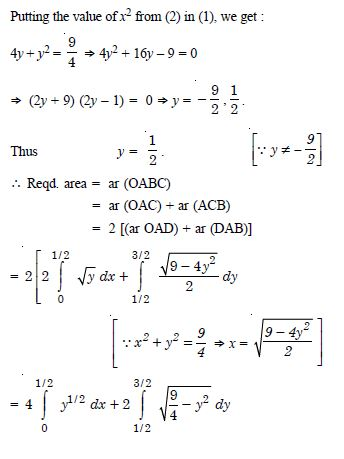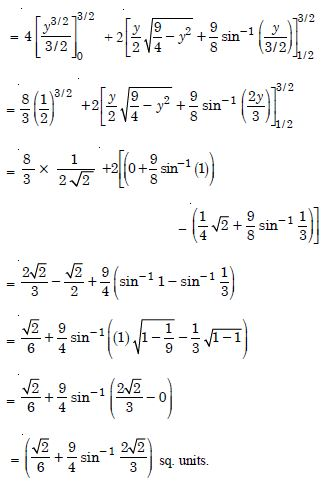##### Question 15:

Find the area bounded by curves :

(x – 1)2 + y2 = 1 and x2 + y2 = 1.

The given circles are :
x2 + y2 = 1 ....(1)
and (x – 1)2 + y2 = 1 ....(2)
(1) is a circle with centre (0, 0) and radius 1.
(2) is a circle with centre (1, 0) and radius 1.
Solving (1) and (2) :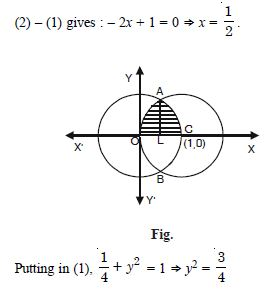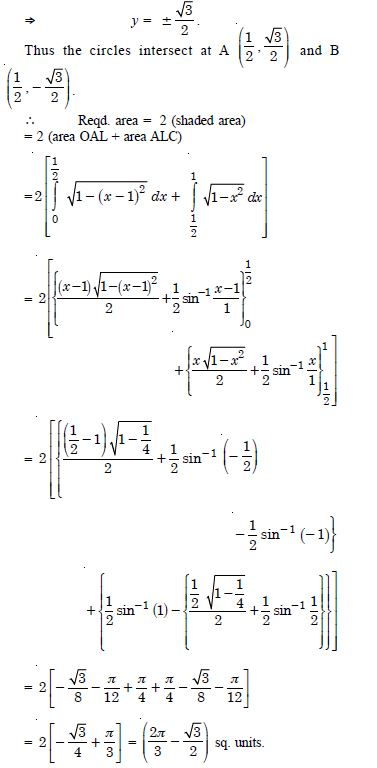##### Question 16:

Find the area bounded by the curves :
y = x2 + 2, y = x, x = 0 and x = 3.

y = x2 + 2 is a parabola.
For tracing, we have the table :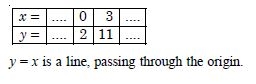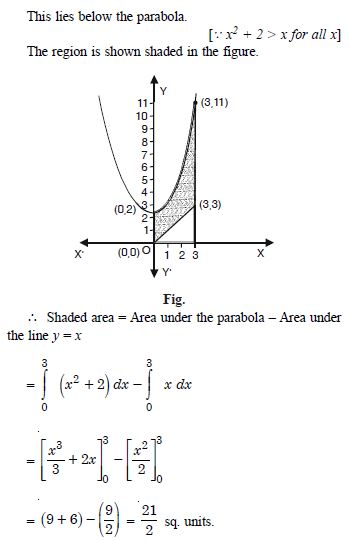##### Question 17:

Using integration, find the area of the region bounded by the triangle whose vertices are :
(– 1, 0), (1, 3) and (3, 2).

Let A (– 1, 0), B (1, 3) and C (3, 2) be the
vertices of the triangle as shown in the figure.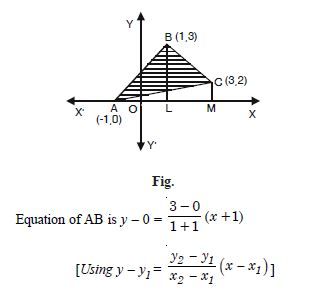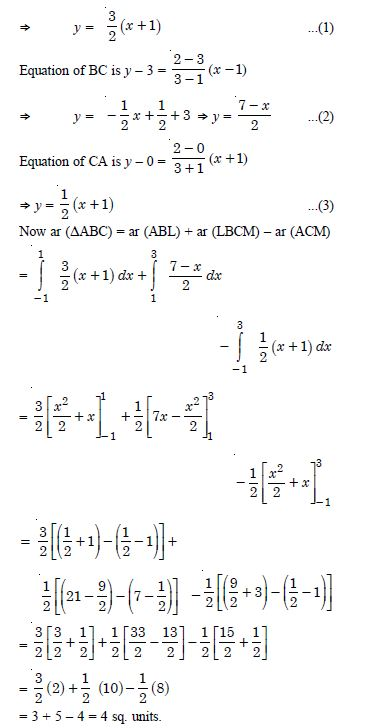##### Question 18:

Using integration, find the area of the triangular region whose sides have the equations :
y = 2x + 1, y = 3x + 1 and x = 4.

The given lines are :
y = 2x + 1 ...(1)
y = 3x + 1 ...(2)
and x = 4 ...(3)
Solving (1) and (2) : we get x = 0, y = 1.
Thus lines (1) and (2) intersect at A (0, 1).
Similarly lines (2) and (3) intersect at B (4, 13)
and lines (3) and (1) intersect at C (4, 9). [Do it]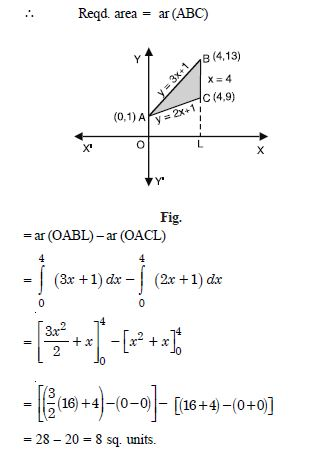##### Question 19:

Smaller area enclosed by the circle x2 + y2 = 4 and the line x + y = 2 is :

1. 2 ($\mathrm{\pi }$ – 2)
2. $\mathrm{\pi }$ – 2
3. 2$\mathrm{\pi }$ – 1
4. 2 ($\mathrm{\pi }$ + 2)

$\mathrm{\pi }$ – 2

##### Question 20:

Area lying between the curves y2 = 4x and y = 2x is :

1.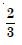2.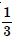3.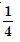4.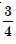##### Question 21:

Area lying in the first quadrant and bounded by the circle x2 + y2 = 4 and the lines x = 0 and x = 2 is :

1. $\mathrm{\pi }$
2.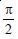3.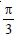4.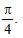$\mathrm{\pi }$

##### Question 22:

Area of the region bounded by the curve y2 = 4x, y-axis and the line y = 3 is :

1. 2
2.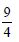3.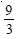4.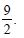##### Question 23:

Smaller area enclosed by the circle x2 + y2 = 4 and the line x + y = 2 is :

1. 2 ($\mathrm{\pi }$ – 2)
2. $\mathrm{\pi }$ – 2
3. 2 $\mathrm{\pi }$ – 1
4. 2 ($\mathrm{\pi }$ + 2)

$\mathrm{\pi }$ – 2

##### Question 24:

Area lying between the curves y2 = 4x and y = 2 is :

1.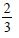2.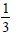3.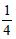4.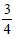##### Question 25:

Area bounded by the curve y = x3, the x-axis and the ordinates x = – 2 and x = 1 is :

1. – 9
2.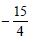3.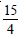4.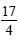##### Question 26:

The area bounded by the curve y = x | x |, x-axis and the ordinates x = – 1 and x = 1 is given by :

1. 0
2.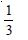3.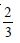4.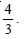##### Question 27:

The area of the circle x2 + y2 = 16 exterior to the parabola y2 = 6x is :

1.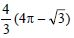2.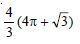3.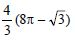4.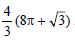##### Question 28:

The area enclosed by the circle x2 + y2 = 2 is equal to :

1. 4$\mathrm{\pi }$ sq. units
2.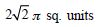3. 4$\mathrm{\pi }$2 sq. units
4. 2$\mathrm{\pi }$ sq. units.

2$\mathrm{\pi }$ sq. units.

##### Question 29:

The area enclosed by the circle x2 + y2 = 2 is equal to :

1. 4$\mathrm{\pi }$ sq. units
2.3. 4$\mathrm{\pi }$2 sq. units
4. 2$\mathrm{\pi }$ sq. units.

2$\mathrm{\pi }$ sq. units.

##### Question 30: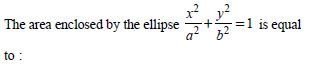1. $\mathrm{\pi }$2ab
2. $\mathrm{\pi }$ ab
3. $\mathrm{\pi }$a2b
4. $\mathrm{\pi }$ab2

$\mathrm{\pi }$ab

##### Question 31:

The area of the region bounded by the curve y = x2 and the line y = 16 is :

1.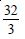2.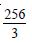3.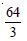4.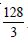##### Question 32: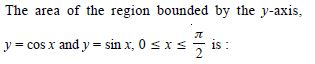1.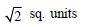2.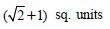3.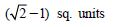4.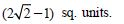##### Question 33:

The area of the region bounded by the curve x2 = 4y and the straight line x = 4y – 2 is :

1.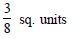2.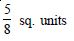3.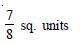4.